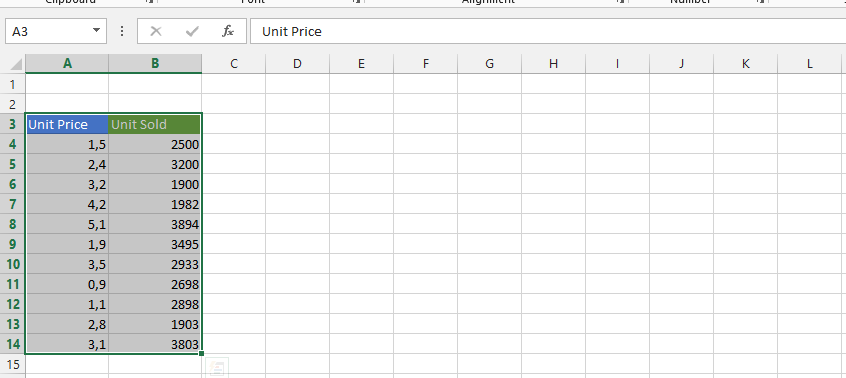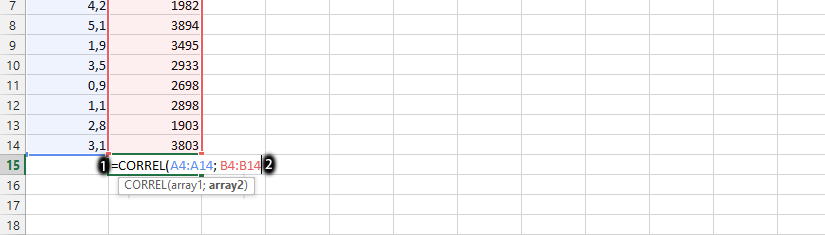#### How to Calculate Mean in Excel

The mean is average of all numbers, regardless how many. We are going to easily calculate it with the help of Excel.

## Calculating a mean

Write all the numbers you want to know the mean of, and laid out in the same roll.To calculate the mean (average) in Microsoft Excel, you can use the AVERAGE function. Click on any blank column and type in =AVERAGE(choose from first to the end ex. A1,A22).Formula here is: =AVERAGE(A1:A14)

Note: The 85.35714 is the mean of all numbers.

See more AVERAGE function examples here.